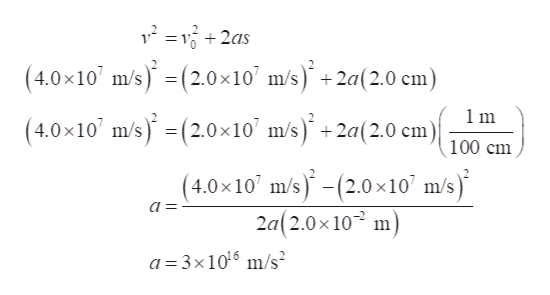An electron moving parallel to a uniform electric field increases its speed from 2.0 × 10^7 m/s to 4.0 × 10^7 m/s over a distance of 2.0 cm.What is the electric field strength? Express your answer with the appropriate units.

Question

An electron moving parallel to a uniform electric field increases its speed from 2.0 × 10^7 m/s to 4.0 × 10^7 m/s over a distance of 2.0 cm.

What is the electric field strength?

Express your answer with the appropriate units.
Step 1

Write the expression of velocity as a function of position.help_outlineImage Transcriptionclose+ (4.0x10 m/s)(2.0x10' m/s) +2a(2.0 cm) (4.0x10 m/s)(2.0x10' m/s+2a(2.0 cm) = 1m 100 cm (4.0x10 m/s)-(2.0x10' m/s) 2a(2.0x 10 m a = a 3x1016 m/s fullscreen
Step 2

The formula for the electric force due to the electric field on the electron is:

Step 3

From Newton’s seco...

Want to see the full answer?

See Solution

Want to see this answer and more?

Our solutions are written by experts, many with advanced degrees, and available 24/7

See Solution
Tagged in

Electric Charges and Fields